# Diffusion equation

(diff) ← Older revision | Latest revision (diff) | Newer revision → (diff)

A second-order partial differential equation describing the process of diffusion, i.e. the process of equalization of the concentration in a medium with an initially non-homogeneous distribution of some substance. The diffusion equation has the form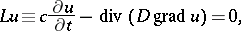(1)

whereis the porosity coefficient,is the diffusion coefficient and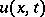is the concentration of the substance at a pointof the medium at the moment of time. The diffusion equation is derived by making up the balance of the substance using Nerst's diffusion law. It is assumed in so doing that sources of the substance and diffusion into an external medium are absent in the domain under consideration. Such a diffusion equation is said to be homogeneous. If the domain under consideration contains sources of the substance with a volume distribution density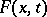, the diffusion process is said to be inhomogeneous with right-hand side. If the substance falls apart or multiplies at a rate proportional to the initial concentration, a termshould be inserted in the right-hand side of the diffusion equation.

The diffusion equation is of parabolic type. In order to find a unique solution, initial and boundary conditions are imposed. The initial condition for a diffusion equation is to specify the concentration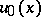of the substance at the initial moment of time: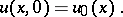(2)

If the substance then fills the entire space, one obtains the Cauchy problem (1), (2). If, on the other hand, the diffusion substance occupies a volumebounded by the side surface, as well as the initial condition (2), a boundary condition is imposed on. The three fundamental linear boundary conditions for a diffusion equation are listed below.

1) The concentration of the substanceis specified on; then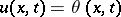is a boundary condition of the first kind.

2) The density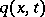of the flow of the substance enteringthroughis given; then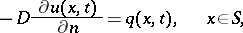whereis the interior normal to the surface, is a boundary condition of the second kind (ifis impermeable,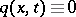).

3) Ifis semi-permeable, and if the diffusion taking place into the external medium with a given concentration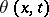throughis linear, thenis a boundary condition of the third kind.

Other boundary conditions, including non-linear ones, may also be imposed on, as well as conditions involving derivatives of a higher order than those which appear in the diffusion equation. Since a diffusion equation is a special case of a differential equation describing physical equalization processes, it is analogous to the thermal-conductance equation, the Navier–Stokes equations for the laminar flow of an incompressible liquid, the equation of pure electric conductance, etc.

How to Cite This Entry:
Diffusion equation. Encyclopedia of Mathematics. URL: http://encyclopediaofmath.org/index.php?title=Diffusion_equation&oldid=17166
This article was adapted from an original article by L.I. Kamynin (originator), which appeared in Encyclopedia of Mathematics - ISBN 1402006098. See original article